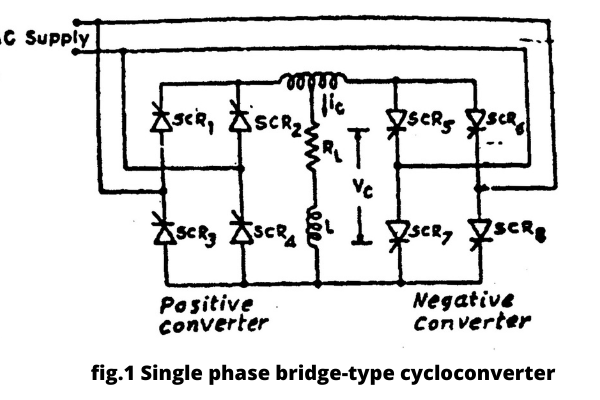# Single Phase Step Down Type Cycloconverter

Hello friends, in today’s article I am going to tell you about that step down bridge type cycloconverter. bridge type cycloconverter, single phase to single phase step down bridge-type cycloconverter: If you also want to know then keep reading this article completely. so let’s start

## bridge type cycloconverter

The circuit of a single-phase to single-phase step-down bridge-type cycloconverter is shown in Figure 1. In this, separate group converters have been taken for positive cycle and negative cycle. In the above circuit, the positive converter provides current to the load in the positive half-cycle and the negative converter provides current to the load in the negative half-cycle.

Due to the danger of a short circuit in the input, both the semi-converters cannot be operated one after the other, so only one semi-converter can provide current to the load at a given time, hence the arrangement of the gate pulse is as follows. It should be done so that only one semi-converter gets a pulse at a time and only when one semi-converter comes into a complete non-operational state, the other semi-converter’s gates should get a pulse. This is called the free circulating mode of the converter.In this mode, the operation can be done by shifting the firing angle. The firing angle control scheme should be periodic according to the output frequency to avoid short-circuiting the input. The firing angles must be the same for both semi-circle converters to produce the same output. Depending on the firing angle and power factor, the load current may be discrete for resistive loads and continuous for inductive loads. For R-L loads, the current lags behind the load voltage, which complicates the operation of the transformers. In Figure 2 the load current lags behind the load voltage. In the region, AB time load voltage is positive, and load current is negative so load current cannot become zero. In this interval, the negative converter should operate with the SCR’s firing angle -α in symbol mode.

In BC time interval the positive converter fires with an angle α. The load current and voltage are positive. The positive converter acts as a rectifier to provide current to the load. The load voltage is opposite in the CD time interval but the load current is still positive. The negative converter must operate in rectifier mode as long as the pulse is received at the firing angle α and the negative converter operates in rectifier mode over the DE time interval and power is supplied to the load.In the time interval ABCD and EF, the instantaneous power is negative and the power is re-invested from the load into the source.

If the sequence of firing angles of both the converters is maintained, the source can be prevented from being short-circuited. This can be done by controlling the gate pulse of the SCR with the load current. Gate pulse control can be somewhat simplified by the flow of the total amount of circulating current. This circulating current can keep both converters hypothetically continuously in the drive state over the entire control range. This operation is called the circulating current mode. In this mode, if the positive converter αp and the negative converter αn are fired at the firing angle then αp + αn = 180°.

In this case, the positive converter is rectified and the negative converter operates in reverse mode. The firing angle of each converter is regulated such that each converter produces the same mean rectified terminal voltage, i.e. the mean value of the voltage generated by the rectifier mode is equal to the mean of the reciprocating force induced in the rectifier mode.

Hence the high-low frequency current between the two group converters can be avoided. But the instantaneous voltage of both the group converters is not the same and heavy consonant current is circulated unless it is reduced by external impedance. The output waveform of a phase bridge converter is given in Fig. 3. In a cycloconverter, the firing angles αp and αn are changed continuously to maintain the relation αp + αn = 180°.Read more: what is filter circuit?

Now you must have known step down bridge type cycloconverter . bridge type cycloconverter, single phase step down bridge-type cycloconverter.